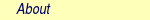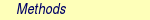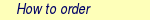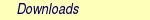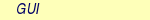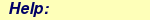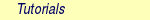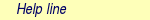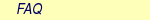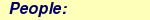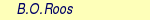MOLCAS manual:Next: 8.28 mclr Up: 8. Programs Previous: 8.26 mbpt2

Subsections

# 8.27 mckinley (a.k.a. denali)

## 8.27.1 Description

This program calculates the second and first order derivatives used for calculating second order derivatives of the energies with perturbation dependent basis sets for SCF and CASSCF wavefunctions. Vibronic frequencies are calculated by the MCLR program which MCKINLEY will start automatically.

McKinley is written such that properties can be computed for any kind of valence basis function that SEWARD will accept. Note, that McKinley can't handle ECP type basis sets.

McKinley is able to compute the following integral derivatives:

• overlap integrals,
• kinetic energy integrals,
• nuclear attraction integrals,
• electron repulsion integrals,
• external electric field integrals,

McKinley employs two different integration schemes to generate the one- and two-electron integral derivatives. The nuclear attraction and electron repulsion integrals are evaluated by a modified Rys-Gauss quadrature . All other integral derivatives are evaluated with the Hermite-Gauss quadrature. The same restriction of the valence basis sets applies as to SEWARD. None of the integral 2nd derivatives are written to disk but rather combined immediately with the corresponding matrix from the wavefunction. Integral 1st derivative information is preprocessed and written to disc for later use in MCLR.

Note that MCKINLEY for frequency calculations normally automatically starts the MCLR with a default input. To override this check the keyword NOMClr.

At present the following limitations are built into McKinley:

 Max number of unique basis functions: 2000 Max number of symmetry independent centers: 500 Highest angular momentum: 15 Highest symmetry point group: D2h

## 8.27.2 Dependencies

McKinley depends on the density, Fock matrices and Molecular orbitals generated by SCF or RASSCF. In addition it needs the basis set specification defined in SEWARD. These dependencies, however, are totally transparent to the user.

## 8.27.3 Files

### 8.27.3.1 Input files

MCKINLEY will use the following input files: RYSRW, ABDATA,RUNFILE (for more information see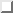).

### 8.27.3.2 Output files

In addition to the standard output unit MCKINLEY will generate the following files.

 File Contents MCKINT File with information needed by the MCLR response program.

## 8.27.4 Input

Below follows a description of the input to MCKINLEY.

The input for each module is preceded by its name like:

&MCKINLEY

Optional keywords
 Keyword Meaning ONEOnly Compile only the nuclear repulsion and one-electron integrals contribution. The default is to compute all contributions. CUTOff Threshold for ignoring contributions follows on the next line. The default is 1.0D-7. The prescreening is based on the 2nd order density matrix and the radial overlap contribution to the integral derivatives. SHOWHessian The Hessian contributions will be printed. PERTurbation This key word takes one of the arguments HESSian or GEOMetry on the following line. HESSian makes MCKINLEY compute the full geometrical Hessian, which is required for a subsequent calculation of the vibronic frequencies with the MCLR program. GEOMetry calculates only those parts of the geometrical Hessian that correspond to symmetry allowed displacements (could be used in a second order geometry optimization). The default is to compute the Hessian for vibrational frequency calculations. Note that in the case of numerical differentiation only symmetry allowed displacements will be computed regardless of this keyword. NOMClr Request that this calculation is not followed by the execution of the MCLR module. VERBose The output will be a bit more verbose.

The following is an example of an input which will work for almost all practical cases.

&MCKINLEY
Perturbation
Hessian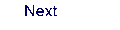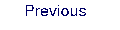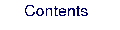Next: 8.28 mclr Up: 8. Programs Previous: 8.26 mbpt2